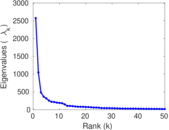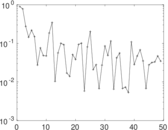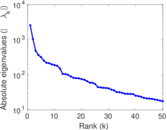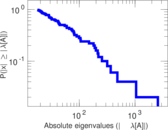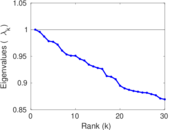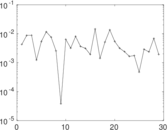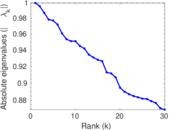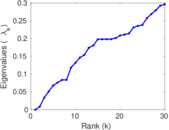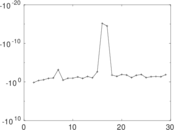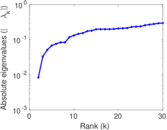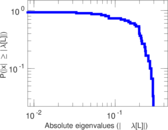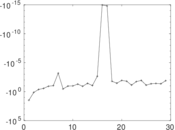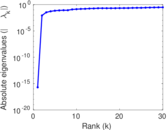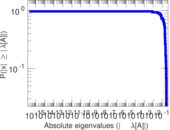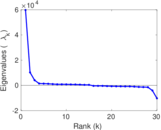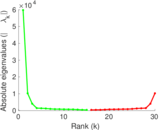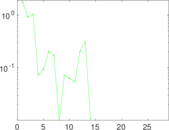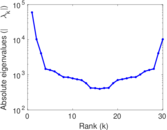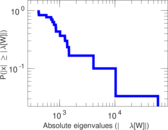# Wiktionary edits (az)

This is the bipartite edit network of the Azerbaijani Wiktionary. It contains users and pages from the Azerbaijani Wiktionary, connected by edit events. Each edge represents an edit. The dataset includes the timestamp of each edit.

 Code `maz` Internal name `edit-azwiktionary` Name Wiktionary edits (az) Data source http://dumps.wikimedia.org/ AvailabilityDataset is available for download Consistency checkDataset passed all tests Category Authorship network Dataset timestamp 2017-10-20 Node meaning User, article Edge meaning Edit Network formatBipartite, undirected Edge typeUnweighted, multiple edges Temporal dataEdges are annotated with timestamps

## Statistics

 Size n = 59,734 Left size n1 = 539 Right size n2 = 59,195 Volume m = 333,151 Unique edge count m̿ = 193,141 Wedge count s = 1,871,434,577 Claw count z = 19,008,224,139,312 Cross count x = 173,764,029,757,213,408 Square count q = 955,760,580 4-Tour count T4 = 15,132,209,542 Maximum degree dmax = 80,773 Maximum left degree d1max = 80,773 Maximum right degree d2max = 3,545 Average degree d = 11.154 5 Average left degree d1 = 618.091 Average right degree d2 = 5.628 03 Fill p = 0.006 053 42 Average edge multiplicity m̃ = 1.724 91 Size of LCC N = 59,342 Diameter δ = 13 50-Percentile effective diameter δ0.5 = 1.754 80 90-Percentile effective diameter δ0.9 = 3.721 93 Median distance δM = 2 Mean distance δm = 2.683 27 Gini coefficient G = 0.729 326 Balanced inequality ratio P = 0.222 401 Left balanced inequality ratio P1 = 0.039 444 6 Right balanced inequality ratio P2 = 0.337 718 Relative edge distribution entropy Her = 0.683 850 Power law exponent γ = 1.991 58 Tail power law exponent γt = 3.731 00 Tail power law exponent with p γ3 = 3.731 00 p-value p = 0.000 00 Left tail power law exponent with p γ3,1 = 1.411 00 Left p-value p1 = 0.018 000 0 Right tail power law exponent with p γ3,2 = 3.871 00 Right p-value p2 = 0.000 00 Degree assortativity ρ = −0.236 358 Degree assortativity p-value pρ = 0.000 00 Spectral norm α = 2,573.62 Algebraic connectivity a = 0.008 368 46 Spectral separation |λ1[A] / λ2[A]| = 2.456 39 Controllability C = 58,590 Relative controllability Cr = 0.982 922

## Plots

### Fruchterman–Reingold graph drawing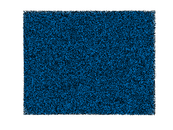### Degree distribution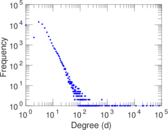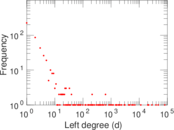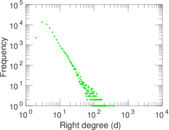### Cumulative degree distribution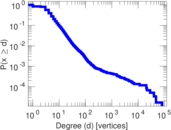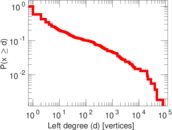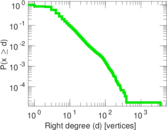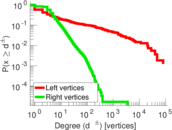### Lorenz curve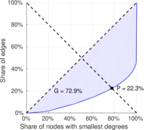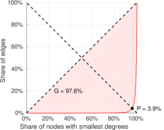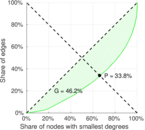### Spectral distribution of the adjacency matrix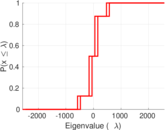### Spectral distribution of the normalized adjacency matrix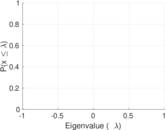### Spectral distribution of the Laplacian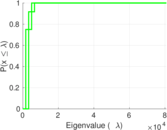### Spectral graph drawing based on the adjacency matrix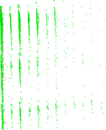### Spectral graph drawing based on the Laplacian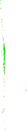### Spectral graph drawing based on the normalized adjacency matrix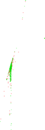### Degree assortativity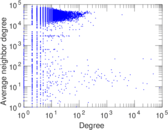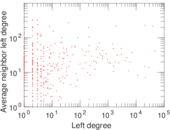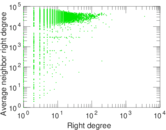### Zipf plot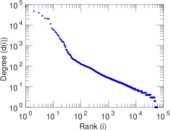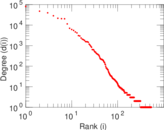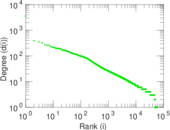### Hop distribution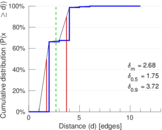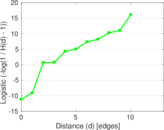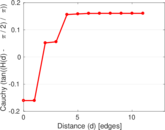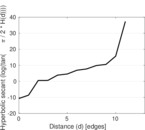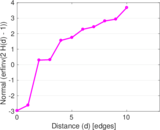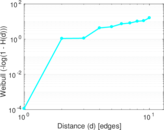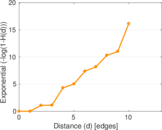### Double Laplacian graph drawing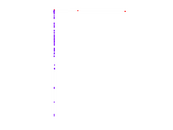### Delaunay graph drawing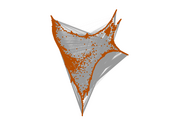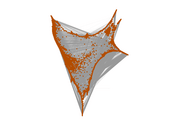### Edge weight/multiplicity distribution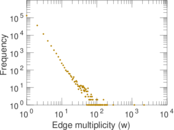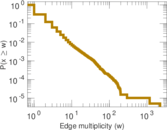### Temporal distribution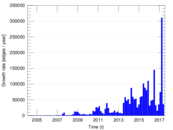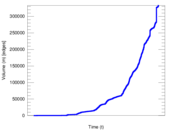### Diameter/density evolution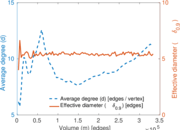### Matrix decompositions plots